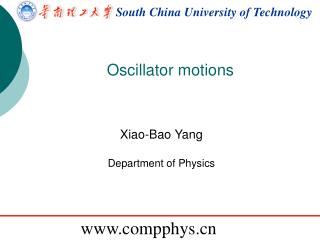DownloadDownload PresentationSouth China University of Technology

# South China University of Technology

Download Presentation## South China University of Technology

- - - - - - - - - - - - - - - - - - - - - - - - - - - E N D - - - - - - - - - - - - - - - - - - - - - - - - - - -
##### Presentation Transcript

1. South China University of Technology Oscillator motions Xiao-BaoYang Department of Physics www.compphys.cn

2. Review of ball trajectory for ii=1:vy(1)/dt*2/g vx(ii+1)=vx(ii); vy(ii+1)=vy(ii)-g*dt; sx(ii+1)=sx(ii)+vx(ii+1)*dt; sy(ii+1)=sy(ii)+vy(ii+1)*dt; end tmp1=sx(ceil(ii/2)+1:end); tmp2=sy(ceil(ii/2)+1:end); tmp3=spline(tmp2,tmp1,bh); if abs(tmp3-d)<0.2 re(iv,ia)=1; end

3. F v wind The effect of spin

4. oscillatory and periodic phenomena the motion of electrons in atoms the behavior of currents and voltages in electronic circuits planetary orbits a pendulum

5. centripetal force and constraint Foucault pendulum

6. Simple Harmonic Motion Euler method

7. Problem with Euler Method clear l=9.8; g=9.8; t(1)=0; theta(1)=0.2; omega(1)=0; dt = 0.04 for i=1:1500; omega(i+1)=omega(i)+dt * (-g/l*(theta(i)); theta(i+1)=theta(i)+omega(i+1)*dt; t(i+1) = t(i) + dt; end Euler-Cromer method Energy not conserved with Euler method. Why?

8. Ordinary Differential Equations with Initial Values (P456) Find the quantity of interest as a function of time. Propagate the relevant function forward in time starting from the given initial value. First order ODE Taylor series Euler method An error of order

9. Alternative way to improve the accuracy Taylor series Iterative approach: Drawback: evaluating partial derivatives of f(x,t) Find proper tm ?

10. Carl Runge The Runge-Kutta method A more practical method that requires only the first point in order to start or to improve the algorithm is the Runge–Kutta method. Determine parameters according to Taylor series: Martin Wilhelm Kutta

11. R-K method in Appendix A

12. R-K method vs Euler method for j=1:nstep; Nu(j+1)=Nu(j)-Nu(j)/tau*dt; %tmp=Nu(j)-Nu(j)/tau*dt/2; %Nu(j+1)=Nu(j)-tmp/tau*dt; t(j+1)=t(j)+dt; end clear Nu(1)=1000; tau=1; dt=0.5; t(1)=0; nstep=20; plot(t,Nu,'o') Nu=1000*exp(-t/tau); hold on plot(t,Nu,'r') plot(t,Nu,'*')

13. Application Illustration! Rk32.m

14. code clear l=1;m=0.1;g=9.8;theta=1./3;omega=0;t(1)=0; t_max=100;dt=0.01;E(1)=.5*m*l^2*(omega(1)^2+g/l*theta(1)^2); for j=1:1000; E(j+1)=.5*m*l^2*(omega(j)^2+g/l*theta(j)^2); d1 = theta(j)+0.5*dt * omega(j); k1 = omega(j)+0.5*dt * (-g/l*theta(j)); theta(j+1)=theta(j)+k1*dt; omega(j+1)=omega(j)+dt*(-g/l*d1); t(j+1)=t(j)+dt; end plot(t,E,'r') plot(t,theta)

15. We can also formally write the solution at t +τas where αi(with i= 1, 2, . . . ,m) and νi j(with i= 2, 3, . . . ,m and j < i) are parameters to be determined. What is the physical meaning of the expansion?

16. Set m=2 2nd Order Runge-Kutta method Now if we perform the Taylor expansion for c2 up to the term O(τ2), we have

17. Typically, there are m Eqs and m + m(m −1)/2 unknowns. 2nd Order Runge-Kutta method E.g., we may choose: Note: These coefficients would result in a modified Euler method …

18. Review of R-K method

19. Application

20. code clear l=1;m=0.1;g=9.8;theta=1./3;omega=0;t(1)=0; t_max=100;dt=0.01;E(1)=.5*m*l^2*(omega(1)^2+g/l*theta(1)^2); for j=1:1000; E(j+1)=.5*m*l^2*(omega(j)^2+g/l*theta(j)^2); d1 = dt * omega(j); k1 = dt * (-g/l*theta(j)); d2 = dt * (omega(j)+k1); k2 = dt * (-g/l*(theta(j)+d1) ); theta(j+1)=theta(j)+(d1+d2)/2.0; omega(j+1)=omega(j)+(k1+k2)/2.0; t(j+1)=t(j)+dt; end

21. 4th Order Runge-Kutta method The well-known fourth-order Runge–Kutta algorithm is given by

22. Making the pendulum more interesting • Adding dissipation illustrations • Adding a driving force • Nonlinear pendulum

23. Homework Exercise 3.1, 3.2, 3.6, • Sending your home work to 17273799@qq.com • Both Results and source codes are required.